## `if` Statement

• An `if` statement tells R to do a certain task for a certain case.
• In English, you would say “If <condition> is true, do <steps>!”
• In R, you would say:
```if (condition) {
step1
step2
...
}```

## `if` Statement

• `condition` object should be a logical statement/test or an R expression that evaluates to a single `TRUE` or `FALSE`.

• If `condition` is `TRUE`,
• R will run all the code that appears between the curly brackets `{}` following the `if` statement.
• If `condition` is `FALSE`,
• R will skip the code between the curly brackets without running it.

## `if` Statement

Below is an example of an `if` statement to make sure a number `num` is positive.

```if (num < 0) {
num <- num * -1
}```

## `if` Statement

• In the case, `num < 0` is `TRUE`:
```num <- -2

if (num < 0) {
num <- num * -1
}

num```
`##  2`

## `if` Statement

• In the case, `num < 0` is `FALSE`:
```num <- 4

if (num < 0) {
num <- num * -1
}

num```
`##  4`

## Quiz 1

• What will the following code return?
```x <- 1
if (3 == 3) {
x <- 2
}
x```

## Quiz 1

• What will the following code return?
```x <- 1
if (3 == 3) {
x <- 2
}
x```
`##  2`

## Quiz 2

• What will the following code return?
```x <- 1
if (TRUE) {
x <- 2
}
x```

## Quiz 2

• What will the following code return?
```x <- 1
if (TRUE) {
x <- 2
}
x```
`##  2`

## Quiz 3

• What will the following code return?
```x <- 1
if (x == 1) {
x <- 2
if (x == 1) {
x <- 3
}
}
x```

## Quiz 3

• What will the following code return?
```x <- 1
if (x == 1) {
x <- 2
if (x == 1) {
x <- 3
}
}
x```
`##  2`

## `else` Statement

• `if` statement tells R what to do when the condition is `TRUE`.
• `else` statement tells R what to do when the condition is `FALSE`.
```if (condition) {
Plan A
} else {
Plan B
}```

## `else` Statement

• Example: Write a function for rounding a number to the nearest whole number.
`a <- 3.14`
• Isolate the decimal component with `trunc()` function.
```decimal <- a - trunc(a)
decimal```
`##  0.14`

## `else` Statement

• Use `if else` to round the number (up or down):
```if (decimal >= 0.5) {
a <- trunc(a) + 1
} else {
a <- trunc(a)
}

a```
`##  3`

## `else` Statement

• Write the rounding function:
```my_round <- function(a) {
decimal <- a - trunc(a)
if (decimal >= 0.5) {
a <- trunc(a) + 1
} else {
a <- trunc(a)
}
return(a)
}```

## `else` Statement

`my_round(3.14)`
`##  3`
`round(3.14)`
`##  3`
`my_round(4.9)`
`##  5`
`round(4.9)`
`##  5`

## `else` Statement

```a <- 1
b <- 1

if (a > b) {
print("A wins!")
} else if (a < b) {
print("B wins!")
} else {
print("Tie.")
}```
`##  "Tie."`

`cars`

## Histogram

`hist(x = cars\$speed)`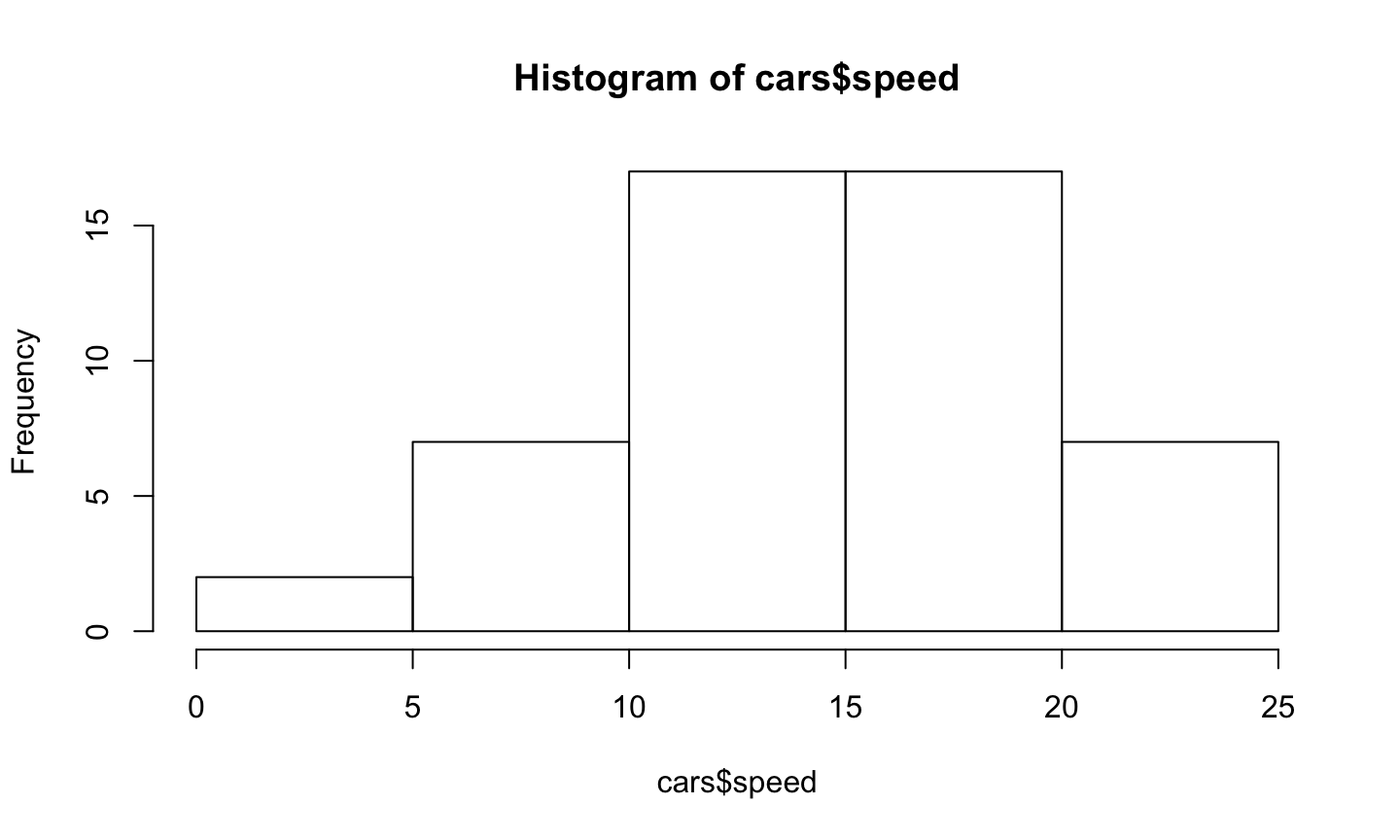## Histogram

• Change the plot title
`hist(x = cars\$speed, main = "Histogram of Speed")`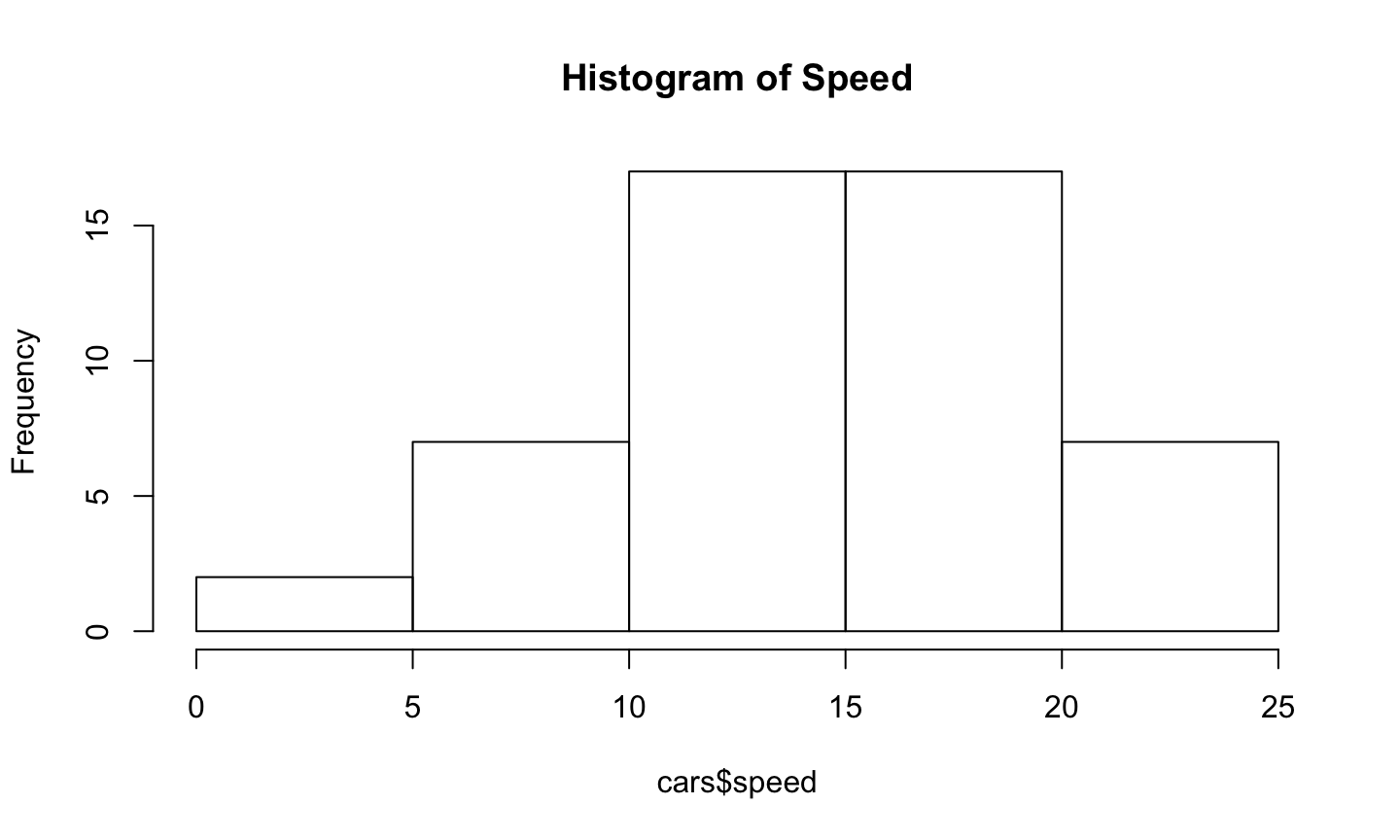## Histogram

• Change x-axis label
`hist(x = cars\$speed, main = "Histogram of Speed", xlab = "Speed (mph)")`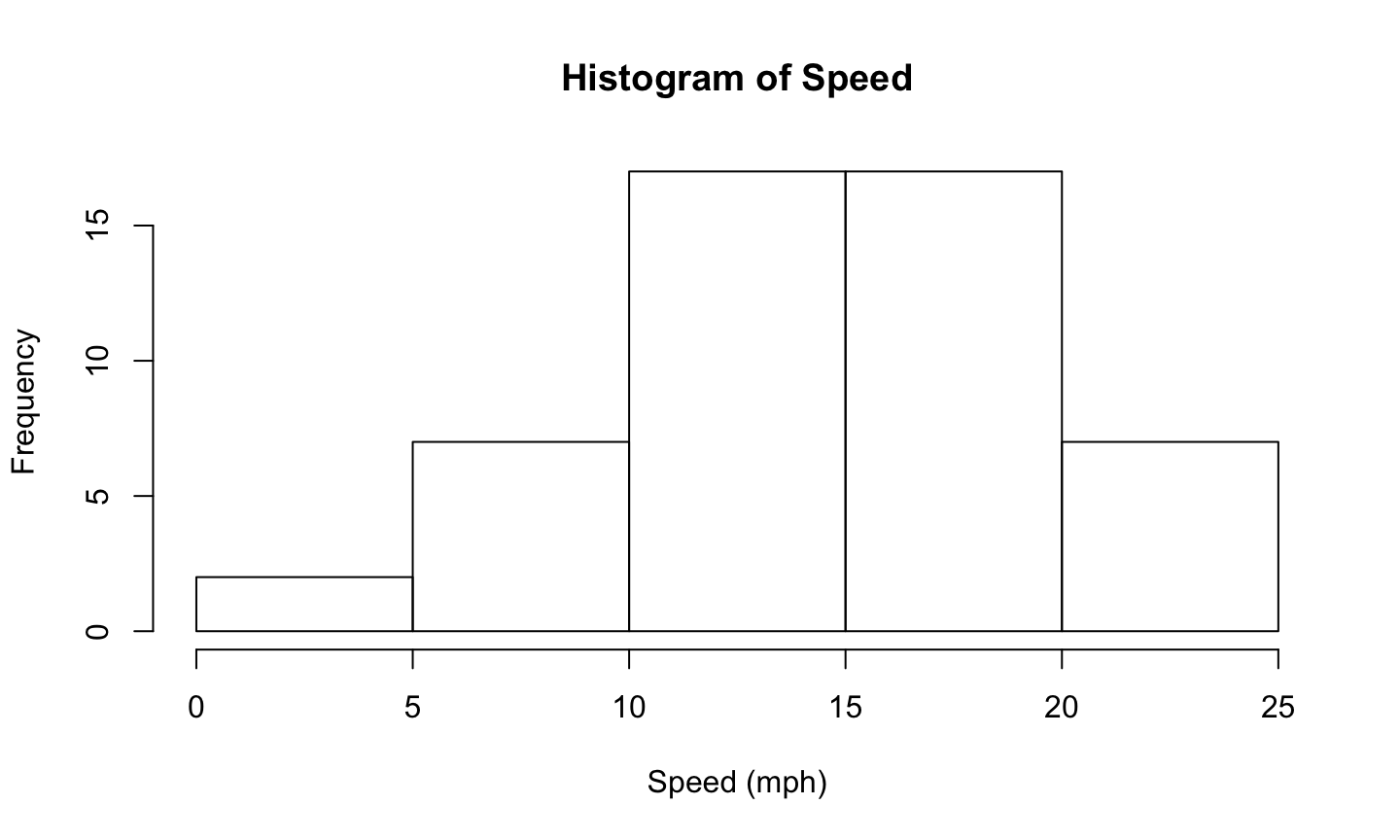## Histogram

• Change the y-axis (from frequency) to probability/density
`hist(x = cars\$speed, main = "Histogram of Speed", xlab = "Speed (mph)", probability = TRUE)`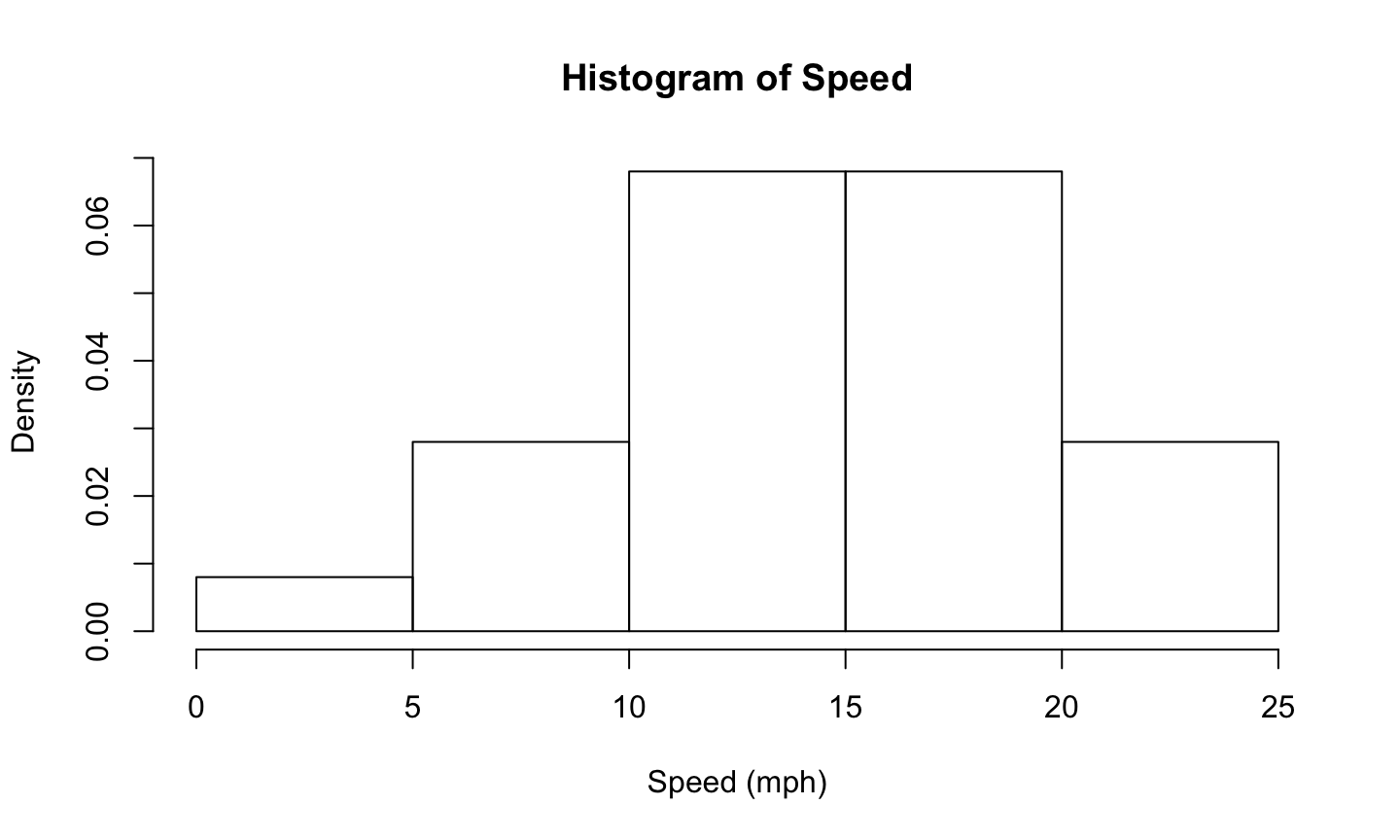## Histogram

• Change how the histogram is graphed (by changing the column width):
• The argument `breaks` in `hist()` can take one of the following:
• a vector giving the breakpoints between histogram cells,
• a function to compute the vector of breakpoints,
• a single number giving the number of cells for the histogram,
• a character string naming an algorithm to compute the number of cells (see ‘Details’),
• a function to compute the number of cells.

## Histogram

```# a vector giving the breakpoints between histogram cells
hist(x = cars\$speed, main = "Histogram of Speed", xlab = "Speed (mph)",
probability = TRUE, breaks = c(0, 4, 8, 12, 16, 20, 24, 28, 32))```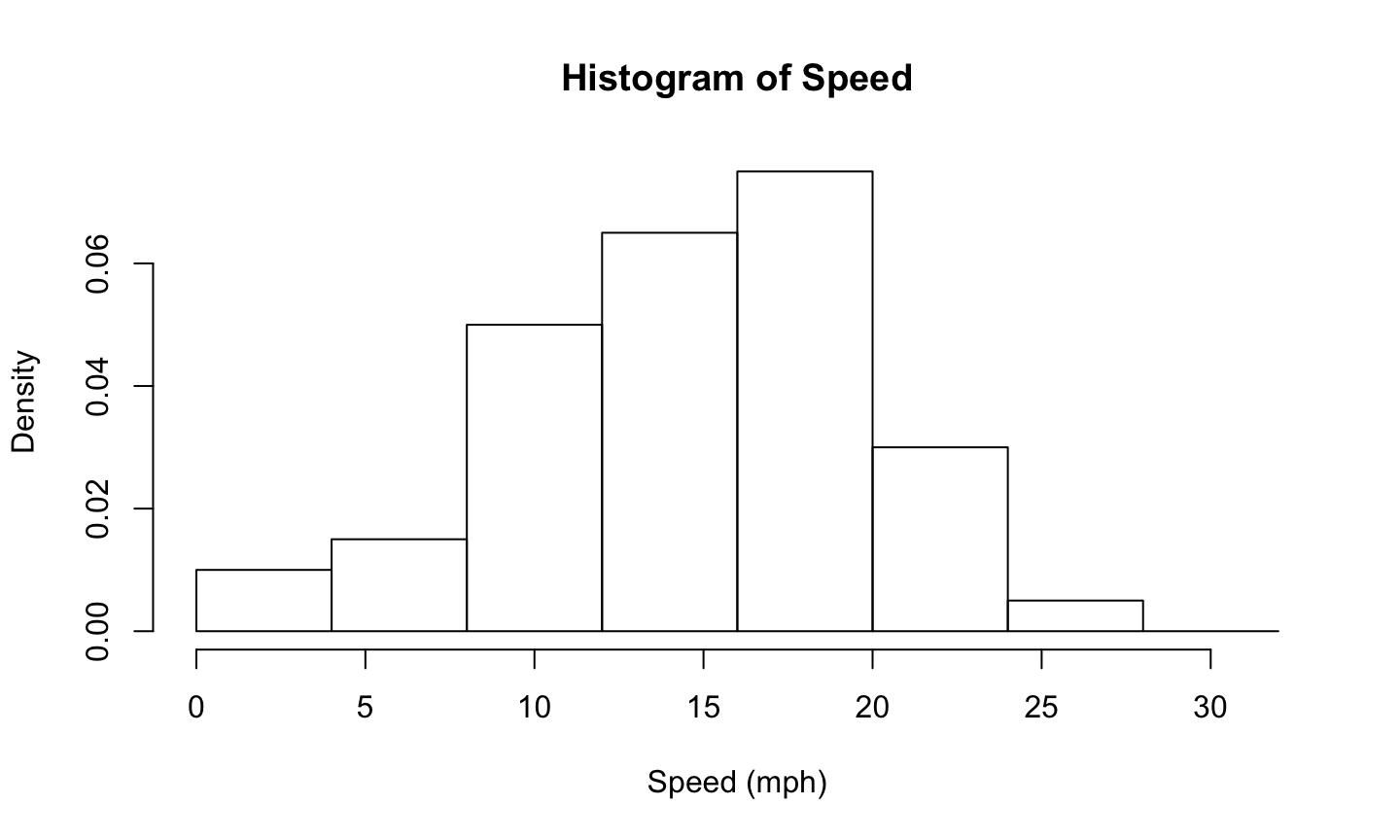## Histogram

```# a function to compute the vector of breakpoints
hist(x = cars\$speed, main = "Histogram of Speed", xlab = "Speed (mph)",
probability = TRUE, breaks = seq(from = 0, to = 30, by = 3))```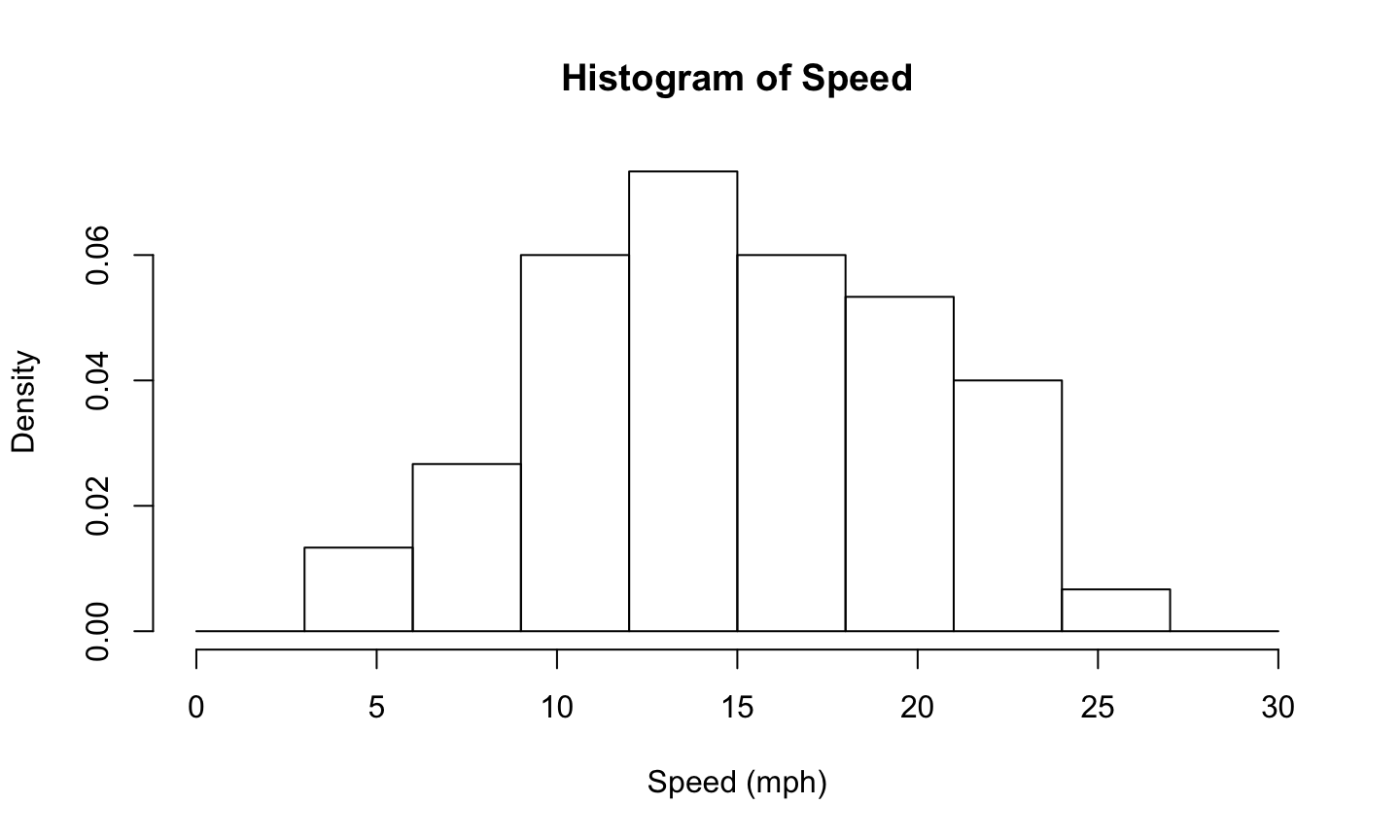## Histogram

```# a single number giving the number of cells for the histogram
hist(x = cars\$speed, main = "Histogram of Speed", xlab = "Speed (mph)",
probability = TRUE, breaks = 10)```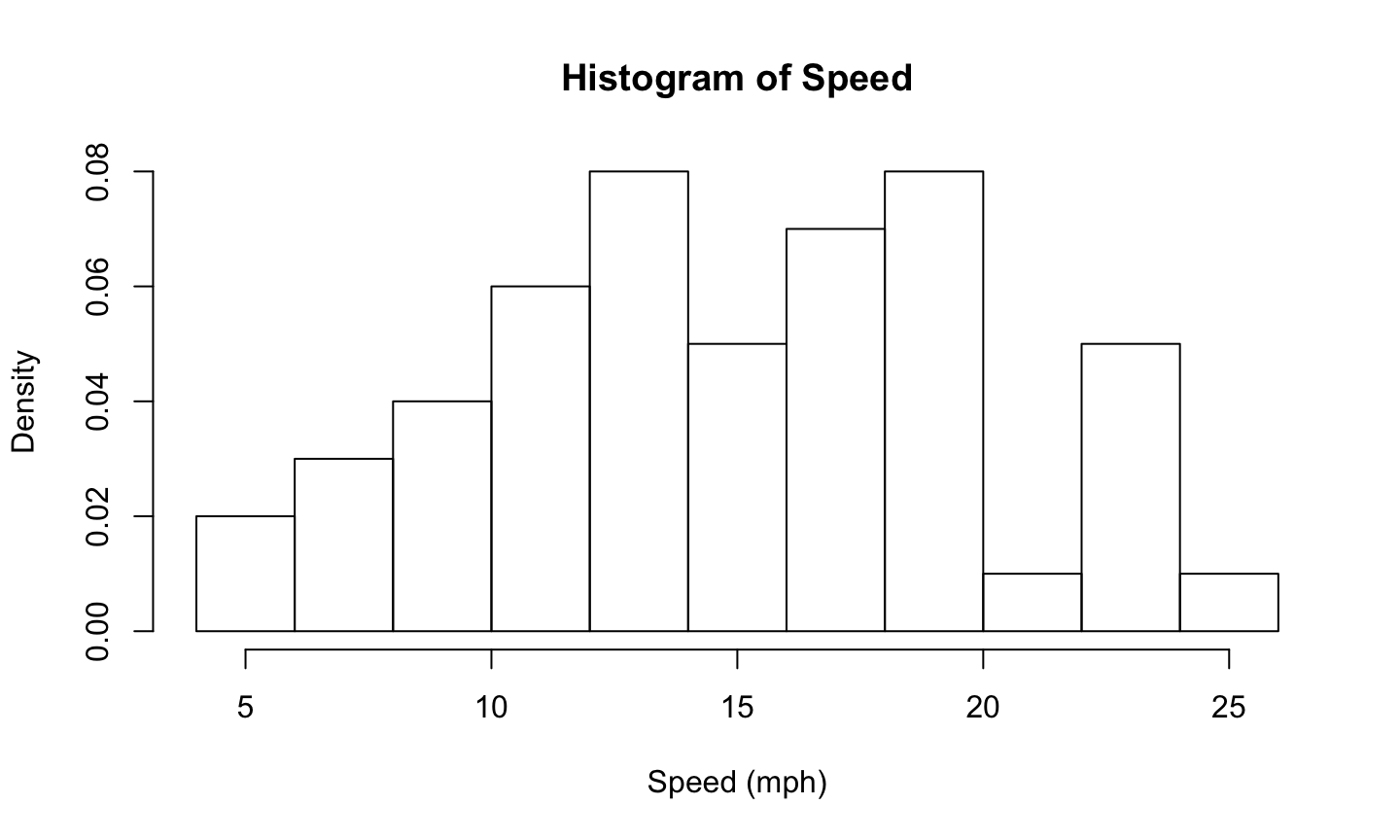## Histogram

```# a single number giving the number of cells for the histogram
hist(x = cars\$speed, main = "Histogram of Speed", xlab = "Speed (mph)",
probability = TRUE, breaks = "Freedman-Diaconis")```## Histogram

```# a function to compute the number of cells
hist(x = cars\$speed, main = "Histogram of Speed", xlab = "Speed (mph)",
probability = TRUE, breaks = 5*2)```